# Why the Circuit Has Low Output Impedance and High Input Impedance?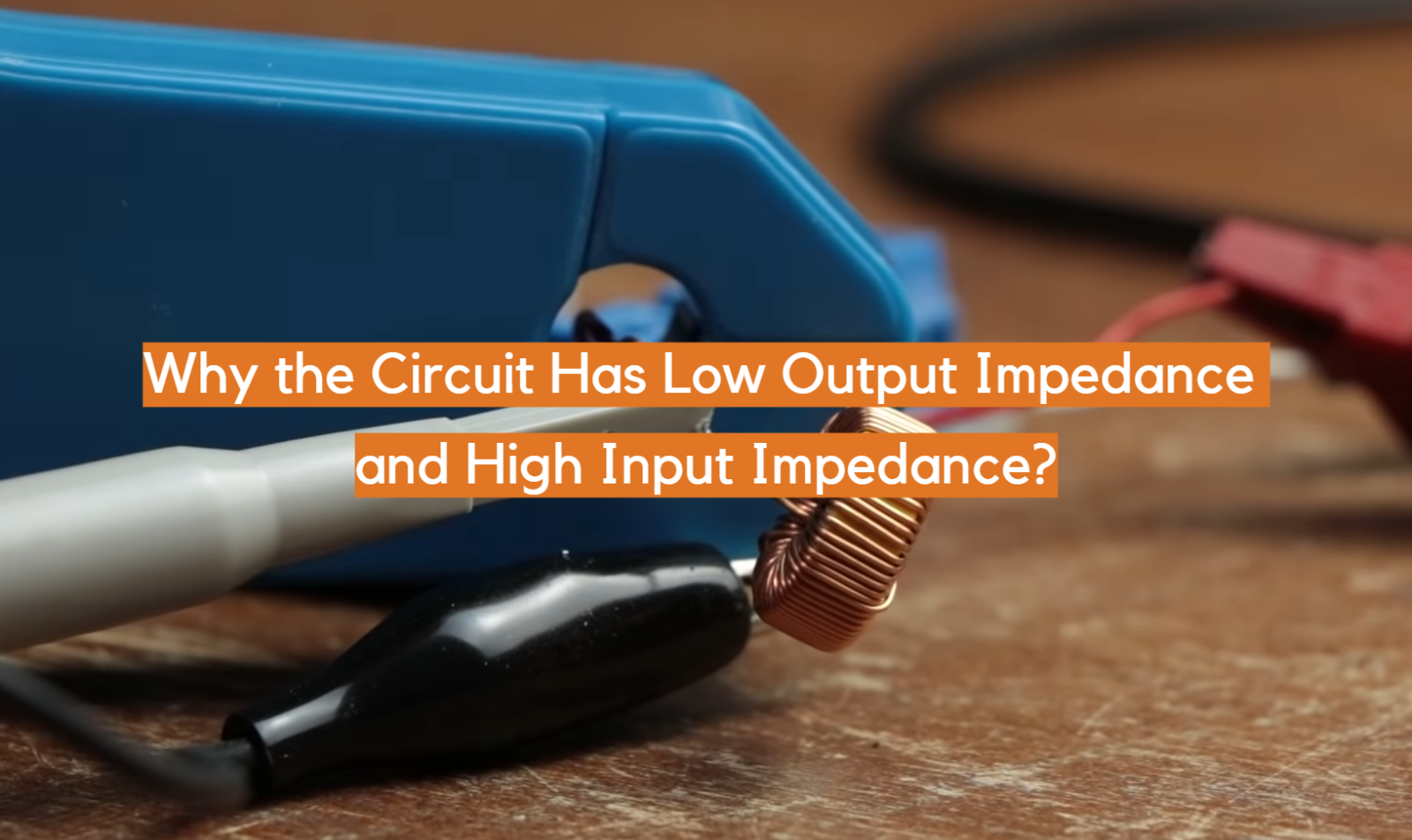In the world of electronic circuits, achieving efficient signal transfer and maintaining signal integrity are fundamental goals. One way to accomplish this is by designing circuits with low output impedance and high input impedance.

This article aims to explore the reasons behind this design choice. Low output impedance ensures efficient power transfer to the load, while high input impedance minimizes the loading effects on the signal source, allowing for accurate signal representation.

Understanding the importance and interplay of these impedance values is essential for designing circuits that perform optimally in terms of power transfer, voltage stability, and signal integrity.

## The Input Impedance and Output Impedance Guide:

### Impedance Overview

Impedance is a measure of the opposition offered by a circuit element to the flow of alternating current (AC). It consists of two components: resistance (R), which hinders the flow of both AC and direct current (DC), and reactance (X), which depends on the frequency of the AC signal. Reactance can be further categorized as capacitive reactance (Xc) or inductive reactance (Xl), depending on the type of component involved .### Input Impedance of Op-Amp

The input impedance of an op-amp refers to the electrical resistance seen at its input terminals. It represents the extent to which the op-amp circuit draws current from the source driving its input. A high input impedance is desirable as it minimizes the loading effect on the signal source, allowing for accurate and efficient signal transfer.

Op-amps typically exhibit extremely high input impedance, often in the range of megaohms (MΩ) or gigaohms (GΩ). This high input impedance ensures that the op-amp draws negligible current from the source, thereby maintaining the integrity of the input signal.

### Output Impedance of Op-Amp

The output impedance of an op-amp refers to the resistance offered by its output terminals to the load connected to it. It signifies the op-amp’s ability to drive current into the load without significant loss or distortion.

A low output impedance is desirable to ensure effective power transfer and accurate voltage amplification. Op-amps generally have low output impedance, typically in the range of tens of ohms (Ω) or less. This low output impedance allows them to deliver signals with minimal loss and maintain voltage stability even when connected to low-impedance loads .### Ideal Op-Amp Impedance

In an ideal scenario, an op-amp would exhibit infinite input impedance and zero output impedance. However, practical op-amps deviate from this ideal behavior due to the limitations of real-world electronic components.

The input impedance of an op-amp is influenced by its internal circuitry, such as the input transistors and biasing elements, while the output impedance is determined by the current output stage of the op-amp. Manufacturers provide specifications for input and output impedance in op-amp datasheets, allowing engineers to select the most suitable op-amp for their specific application requirements.

### High Input Impedance and Low Output Impedance Effect

The high input impedance of an op-amp ensures that it draws minimal current from the signal source, preventing loading effects that could distort the input signal. This characteristic is particularly crucial when interfacing with high-impedance sources, such as sensors or microphones, as it allows for accurate signal representation and prevents signal degradation.

The low output impedance of an op-amp allows it to drive current into low-impedance loads without significant voltage drop. This capability is essential when connecting the op-amp output to subsequent stages or external devices, as it ensures efficient signal transmission and minimizes signal degradation.

### How to Calculate Input Impedance and Output Impedance

Calculating the input impedance of an op-amp requires analyzing the equivalent input circuit of the op-amp and considering the impedance contributed by each component. This analysis involves understanding the internal circuitry of the op-amp and its behavior at the input terminals. Different op-amp models may have variations in their internal circuitry, so it is important to consult the datasheet and manufacturer’s specifications for accurate calculations .

The output impedance of an op-amp can be determined by analyzing the output circuitry and taking into account the impedance contributed by the active components, such as transistors and resistors. Again, referring to the op-amp datasheet and understanding the specific model’s characteristics is essential for accurate calculations.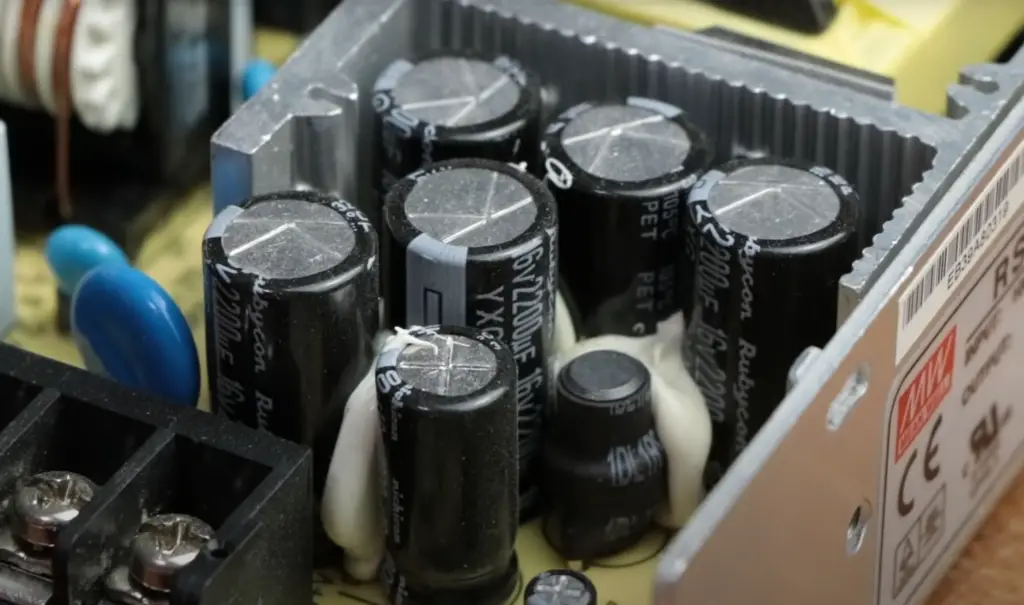## Why Does The Circuit Have Low Output Impedance And High Input Impedance?

### Low Output Impedance

A low output impedance means that the circuit can effectively drive current into a load without significant voltage drop. It is essential when connecting the circuit to subsequent stages or external devices.

Here are a few reasons why low output impedance is desired:

• Power Transfer Efficiency: When the output impedance is low, the circuit can transfer power more efficiently to the load. This is particularly important when driving low-impedance loads, such as speakers or antennas, where the load impedance may be significantly smaller than the output impedance. By minimizing the voltage drop across the output impedance, the circuit can deliver a substantial portion of the available power to the load;
• Voltage Stability: A low output impedance helps maintain voltage stability even when connected to varying loads. When a load with a lower impedance is connected to a circuit, the load tries to draw more current. If the output impedance is high, this increased current flow can cause a significant voltage drop across the output impedance, leading to signal distortion and loss. However, with a low output impedance, the circuit can effectively counteract the load’s impedance, ensuring that the voltage remains stable and the signal integrity is preserved;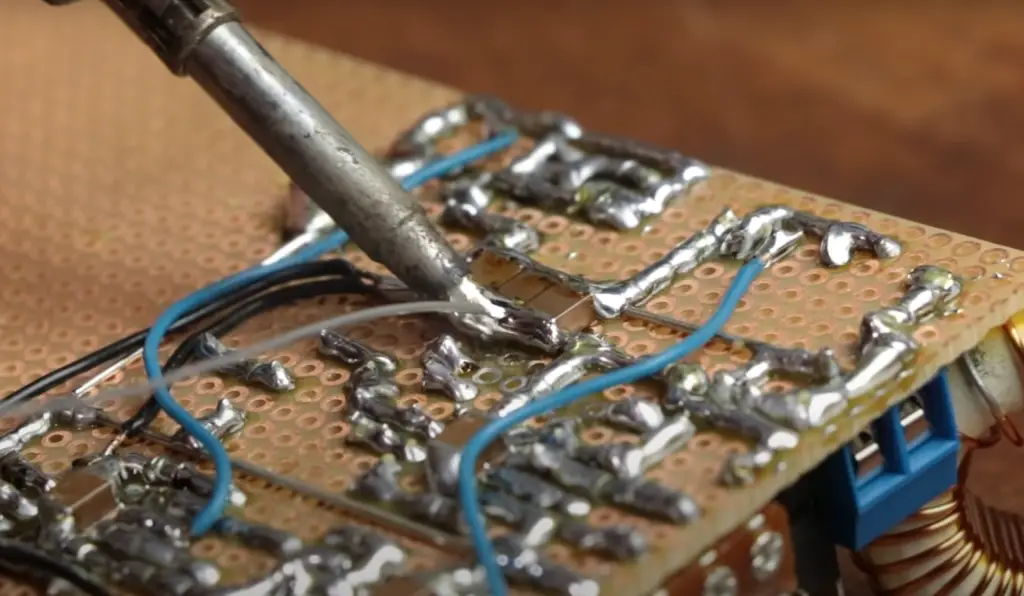### High Input Impedance

High input impedance refers to the circuit’s ability to draw minimal current from the source driving its input. It is crucial for accurate signal representation and preventing loading effects.

Here are a few reasons why high input impedance is desirable:

• Signal Source Integrity: When connecting a signal source to a circuit, such as a sensor or a microphone, it is essential to minimize the loading effect on the source. A high input impedance ensures that the circuit draws negligible current from the source, preventing any significant voltage drop across the source’s internal impedance. This allows the signal source to operate in its optimal voltage range and ensures accurate signal representation;
• Minimized Signal Degradation: High input impedance prevents the signal source from being excessively loaded, which can lead to signal degradation. When the input impedance is low, it draws more current from the source, which can affect the source’s output voltage and cause distortion. By having a high input impedance, the circuit minimizes the current drawn, preserving the source’s output voltage and minimizing signal degradation;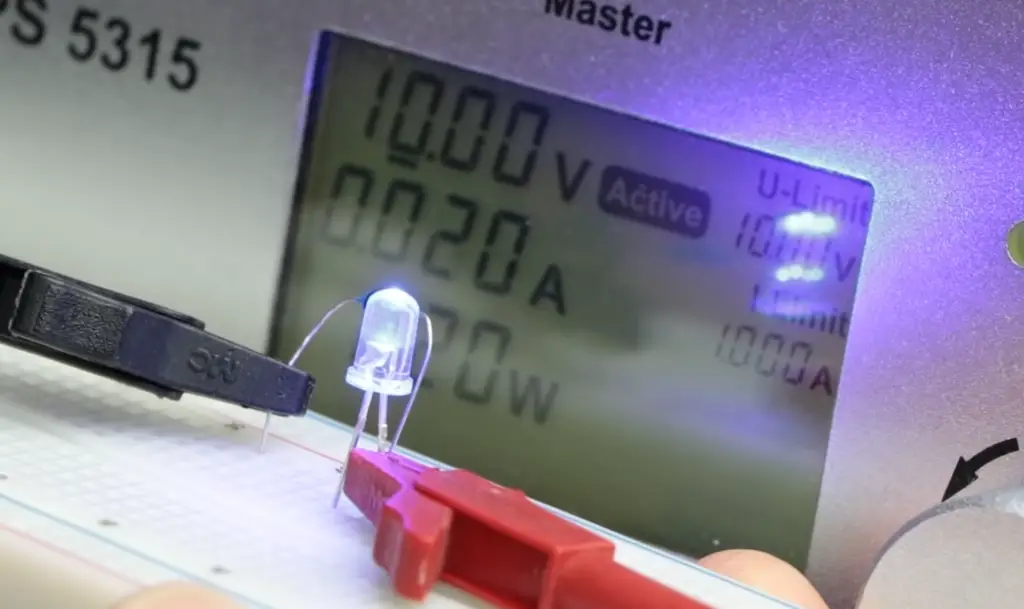## Why Does an Op-Amp Have a High Input Impedance and a Low Output Impedance?

The op-amp has a high input impedance and a low output impedance for two main reasons:

• Firstly, an op-amp is designed to amplify the signal without introducing any additional loading that would cause the signal’s amplitude to drop. To accomplish this, the op-amp must have a very high input resistance so that it does not draw current from its inputs and therefore does not reduce the amplitude of the applied signal;
• The second reason is related to feedback circuitry; having a low output impedance, it allows for more efficient negative feedback by ensuring that most of the output voltage appears across the load instead of being lost in internal resistances. This helps ensure accurate control signals even at large gains ;

Having a low output impedance also allows the op-amp to drive higher current loads than it would otherwise.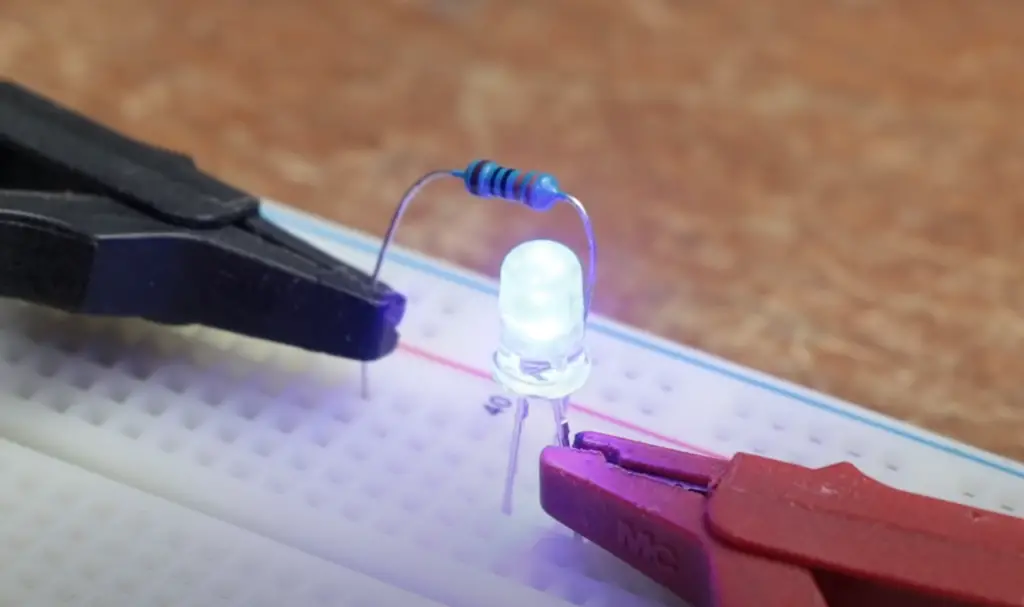## FAQ:

### 1. Why is output impedance low?

Output impedance is designed to be low to ensure efficient power transfer from the circuit to the load. A low output impedance minimizes the voltage drop across the impedance itself, allowing a significant portion of the available power to be delivered to the load. This is crucial when driving low-impedance loads to maintain voltage stability and minimize signal distortion.

### 2. What causes high input impedance?

High input impedance is typically achieved by designing the circuit with components that offer minimal current flow into the input terminals. The use of high-value resistors or field-effect transistors (FETs) in the input stage of a circuit can contribute to high input impedance. By limiting the current drawn from the source driving the input, high input impedance ensures accurate signal representation and prevents loading effects.

### 3. What is the relation between input impedance and output impedance?

Input impedance and output impedance are two distinct characteristics of a circuit. Input impedance refers to the resistance seen by the signal source driving the input, while output impedance refers to the resistance offered by the circuit to the load connected to its output.

The relationship between the two is that a circuit with high input impedance and low output impedance is desirable for efficient signal transfer and minimal signal degradation.

### 4. What is the effect of input and output impedance?

Input impedance affects the signal source by minimizing the loading effect and allowing accurate signal representation. It ensures that the source operates in its optimal voltage range. Output impedance affects the connected load by determining the efficiency of power transfer and voltage stability. A low output impedance ensures efficient power delivery and minimal voltage drop across the impedance.

### 5. Is high impedance input or output?

High impedance is typically associated with the input stage of a circuit. It refers to the resistance seen by the signal source driving the input terminals. High input impedance minimizes the current drawn from the source and prevents loading effects.

### 6. Why is higher impedance better?

Higher impedance is often better because it allows for minimal current flow, reducing loading effects on the source and ensuring accurate signal representation. It also provides improved isolation between different parts of a circuit and allows for effective power transfer to low-impedance loads.

### 7. What happens if the impedance is too high?

If the impedance is too high, it can result in reduced signal strength and poor power transfer. Excessively high impedance can cause voltage drop and distortion in the signal, leading to signal degradation and loss of information. Additionally, if the source driving the high impedance cannot supply enough current, the voltage may drop significantly, resulting in a weak or distorted signal.

### 8. Is low impedance better than high impedance?

The ideal impedance value depends on the specific requirements of the circuit and the nature of the signal source and load. Low impedance is generally better for efficient power transfer and voltage stability, while high impedance is preferred to minimize loading effects on the source and ensure accurate signal representation. The choice between low and high impedance depends on the specific application and desired performance.

### 9. Why is the output impedance 50 ohm?

The choice of 50 ohms as a standard output impedance value is primarily related to the transmission of high-frequency signals in communication systems. A 50-ohm output impedance matches the characteristic impedance of many transmission lines and cables used in these systems, allowing for optimal signal transfer and minimal signal reflections.

### 10. Why is lower impedance better?

Lower impedance is generally better because it allows for efficient power transfer, minimal voltage drop, and improved voltage stability. It enables the circuit to deliver a significant portion of the available power to the load without significant signal degradation.

### 11. How does impedance affect voltage?

Impedance affects voltage by influencing the voltage drop across a circuit element. When current flows through an impedance, a voltage drop occurs according to Ohm’s law (V = I * Z), where V is the voltage drop, I is the current, and Z is the impedance. Higher impedance results in a larger voltage drop for the same current.

### 12. Why does input impedance matter?

Input impedance matters because it determines the loading effect on the signal source and influences the accuracy of signal representation. A high input impedance ensures minimal current flow from the source, preventing signal degradation and maintaining the source’s voltage within its optimal range.

### 13. Which has high input impedance?

Circuits or components designed with high-value resistors or FETs in their input stage typically have high input impedance. Examples include instrumentation amplifiers, buffer amplifiers, and input stages of operational amplifiers.

### 14. What is an example of high impedance?

A piezoelectric sensor, such as those used in acoustic or vibration measurements, typically has high impedance. These sensors generate very small currents in response to the physical phenomena they measure, and their high impedance allows for accurate signal representation and prevents loading effects.

### 15. Why is impedance important in a circuit?

Impedance is important in a circuit as it influences the behavior of the circuit with respect to current and voltage. It determines the power transfer efficiency, voltage stability, and accuracy of signal representation. Understanding and managing impedance is crucial for designing circuits that perform optimally in terms of signal integrity and power transfer.

### 16. Why does output impedance matter?

Output impedance matters because it determines the ability of a circuit to drive current into a load without significant voltage drop. A low output impedance ensures efficient power transfer, voltage stability, and minimal signal distortion when connected to subsequent stages or external devices.

### 17. Why is input impedance infinite?

In ideal theoretical models, the input impedance can be considered infinite to avoid drawing any current from the signal source. However, practical circuits have finite input impedance due to limitations imposed by real-world components and design considerations.

### 18. Does Ohm’s law apply to impedance?

Yes, Ohm’s law applies to impedance. Ohm’s law states that the voltage across an impedance is proportional to the current flowing through it, with the impedance value determining the proportionality constant. Mathematically, V = I * Z, where V is the voltage, I is the current, and Z is the impedance.

### 19. Does impedance increase or decrease?

Impedance can increase or decrease depending on the circuit elements and their arrangement. Adding resistors, capacitors, or inductors in series or parallel can affect the total impedance of a circuit. For example, inductive elements increase impedance with frequency, while capacitive elements decrease impedance with frequency.

### 20. What affects impedance?

Impedance is affected by the characteristics and arrangement of circuit elements such as resistors, capacitors, and inductors. The values of these components, as well as their frequency-dependent properties, influence the overall impedance of a circuit.

### 21. Does higher impedance mean more power?

No, higher impedance does not necessarily mean more power. Power is determined by the product of voltage and current (P = V * I) . In a circuit, power transfer efficiency increases with lower impedance, as more power can be delivered to the load without significant voltage drop across the impedance.

### 22. What is the effect of low impedance?

Low impedance allows for efficient power transfer, minimal voltage drop, and improved voltage stability. It enables the circuit to deliver a significant portion of the available power to the load without significant signal degradation.

### 23. Why do we use 50 ohm and 75 ohm resistors in cables?

The choice of 50 ohms and 75 ohms for resistors in cables is related to their characteristic impedance. These values match the characteristic impedance of specific types of transmission lines and cables used in communication systems, ensuring optimal signal transfer, minimizing signal reflections, and maintaining signal integrity.

### 24. What is the difference between impedance and resistance?

Resistance refers to the opposition to the flow of electric current in a circuit, while impedance encompasses both resistance and reactance.

Impedance accounts for the phase relationship between current and voltage in circuits that involve reactive components such as capacitors and inductors.

### 25. How many ohms is high impedance?

The threshold for what is considered high impedance depends on the specific application and context. In general, an impedance above several hundred kilohms or even megohms can be considered high in many electronic circuits .

### 26. What increases impedance?

Impedance can be increased by adding resistors, inductors, or capacitors in series or parallel. The values and arrangement of these components influence the overall impedance of a circuit.

### 27. How does impedance affect the signal?

Impedance affects the signal by influencing the voltage drop and power transfer efficiency. A mismatch between the impedance of a signal source and a load can cause signal reflections, distortions, and signal degradation. Proper impedance matching ensures optimal signal transfer and maintains signal integrity.

### 28. Does high impedance mean an open circuit?

No, high impedance does not necessarily mean an open circuit. While an open circuit has infinite impedance, a high-impedance circuit allows for some current flow while minimizing loading effects on the signal source.

### 29. What happens if the input impedance is too low?

If the input impedance is too low, it can cause excessive current flow from the signal source, leading to significant voltage drop and distortion. It can also overload the source, affecting its performance and potentially damaging it.

### 30. Which connection decreases both input and output impedance?

The connection of an impedance-matching transformer can decrease both input and output impedance. This transformer matches the impedance of the source to that of the load, ensuring optimal power transfer and maintaining signal integrity.